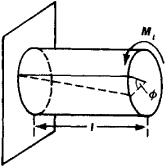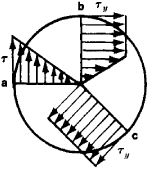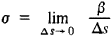# torsion

Also found in: Dictionary, Thesaurus, Medical, Acronyms, Wikipedia.
Related to torsion: torsion bar, Torsion of testis

## torsion,

stress on a body when external forces tend to twist it about an axis. See strength of materialsstrength of materials,
measurement in engineering of the capacity of metal, wood, concrete, and other materials to withstand stress and strain. Stress is the internal force exerted by one part of an elastic body upon the adjoining part, and strain is the deformation or change in
.

## Torsion

The force tending to twist an architectural member.

## Torsion

(in strength of materials), a type of deformation characterized by rotation of the cross sections of a rod or shaft relative to one another under the influence of moments (force couples) acting in them. Upon torsion, the cross sections of round rods or shafts remain plane; upon torsion of a prismatic bar, warping of the cross section takes place (it does not remain plane). If the warping is different in various sections, then normal stresses also arise in the cross sections of the rod, in addition to tangential stresses. In this case the torsion is called constrained. In free torsion, in which the warping in all sections is identical, only tangential stresses arise in the cross section.

In practice, the most common type of torsion is torsion of a round, straight rod (Figure 1). As a result of the torsion moment Mt tangential stresses τ arise in the cross sections of the rod, and the rod segments (separated by distance l) are rotated relative to each other by an angle of twist ϕ. The angle of twist per unit length of the rod is called the relative angle of twist Θ. In free torsion, the relative angle of twist and the maximum tangential stresses τmax in the elastic phase are found from the formulas

θ = Mt/GIt τmax = Mt/Wt

where G is the elastic shear modulus and It and Wt are the arbitrary moment of inertia and the sectional modulus of torsion. In round sections, It is a polar moment of inertia Ip = πr4/2, and Wt is a polar moment of resistance Wp = πr3/2. For rectangular sections with a greater side h and lesser side b, It = αhb3 and Wt = βhb2, where the coefficients α and β are determined according to the ratio h/b from a table. For narrow sections (h/b ≧ 10), it is possible to take α = β ≈ 1/3.Figure 1. Torsion of a round shaft fastened at one end

For torsion of a round shaft in the elastic phase, the tangential stresses are distributed linearly throughout the cross section (Figure 2,a) and are determined by the formula τ = (Mt/ Iρ, where ρ is the distance from the axis of the shaft to the point of the section in question. In the elastic-plastic phase the torsional tangential stresses corresponding to the yield limit τy propagate from the surface toward the axis of the shaft (Figure 2,b). In the limiting state, the plastic zone extends to the axis of the shaft (Figure 2,c), and the limiting tortion moment for a round section is

Mlim = 2/3 (τyπr3)

The concept of torsion has also been extended to the action of internal tangential stresses arising during deformation of plates and casings.Figure 2. Distribution of tangential stresses in the cross section of a round shaft: (a) in the elastic phase, (b) in the elastic-plastic phase, (c) in the plastic phase

### REFERENCES

Beliaev, N. M. Soprotivlenie materialov, 12th ed. Moscow, 1959.
Kurs soprotivleniia materialov, 5th ed., part 1. Moscow, 1961.
Novozhilov, V. V. Teoriia uprugosti. Leningrad, 1958.

L. V. KASAB’IAN

## Torsion

(mathematics), the measure of the deviation of a space curve from its osculating plane. The torsion of a curve at the point M is determined in the following manner. Let N be a variable point on a curve sufficiently close to M and let β be the acute angle between the osculating planes at M and N. The angle β is considered to be positive if as N approaches M a variable osculating plane appears to an observer looking from M to N to rotate in the counterclockwise direction; in the opposite case the angle β is considered to be negative. The limit of the ratio β/ΔS—where ΔS is the arc length MN —as N approaches M is called the torsion < of the curve at the point M:(See.)

## torsion

[′tȯr·shən]
(mathematics)
The rate of change of the positive direction of the binormal of a space curve with respect to arc length along the curve; its sign is defined as positive if it is in the same direction as the principal normal, and negative if it is in the opposite direction. Also known as second curvature.
(mechanics)
A twisting deformation of a solid body about an axis in which lines that were initially parallel to the axis become helices.

## torsion

The twisting of a structural member about its longitudinal axis by two equal and opposite torques, one at one end and the other at the opposite end.

## torsion

a. the twisting of a part by application of equal and opposite torques at either end
b. the condition of twist and shear stress produced by a torque on a part or component
References in periodicals archive ?
Omental torsion can simply be defined as twisting of the omentum on itself especially on its long axis.
C[O.sub.2] laser radiation-engraved gratings exhibit special features that allow the fabrication with very short lengths and explain their torsion sensitivity and torsion sense discrimination.
Ten percent (48) of patients underwent emergency surgery because testicular torsion was suspected.
In keeping with the current reverence for that very aesthetic, the new Torsion TRDC is a painstakingly faithful update of the silhouette, materials and technologies that makes the original such a lost gem of the adidas back catalogue.
In the first semester, the bud burst percentage in the plants in which the torsion of canes was performed was superior to that of the plants without the torsion (Table 1), with a better bud fertility, increasing in 12.56% the bud burst rate.
In a review article by Sells et al, (24) recurrence of torsion after fixation was found to be more common in cases where absorbable sutures were used compared to cases in which non-absorbable sutures were used.
Testicular torsion is the result of spermatic cord twisting causing decreased perfusion to the testicle.
The first method studied is to increase the part thickness on chassis frame to improve the torsion stiffness.
Omental torsion often occurs in the 4th and 5th decades and usually in males.
No torsion was observed in double Maddox rod test and fundus images.
Experiments conducted to evaluate the effect of torsion on an aluminum alloy and pure copper, by Horita and Langdon , showed that significant grain refinement is achieved in both materials with grain sizes of 150 and 140[micro]m, respectively.

Site: Follow: Share:
Open / Close